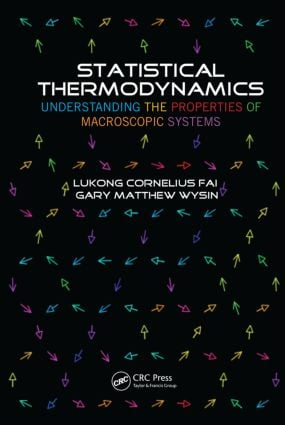# Statistical Thermodynamics

## Understanding the Properties of Macroscopic Systems, 1st Edition

CRC Press

548 pages | 83 B/W Illus.

Paperback: 9780367380762
pub: 2019-07-31
SAVE ~\$14.99
Available for pre-order
\$74.95
\$59.96
x
Hardback: 9781466510678
pub: 2012-10-19
SAVE ~\$19.59
\$97.95
\$78.36
x
eBook (VitalSource) : 9780429086748
pub: 2012-10-19
from \$48.98

FREE Standard Shipping!

### Description

Statistical thermodynamics and the related domains of statistical physics and quantum mechanics are very important in many fields of research, including plasmas, rarefied gas dynamics, nuclear systems, lasers, semiconductors, superconductivity, ortho- and para-hydrogen, liquid helium, and so on. Statistical Thermodynamics: Understanding the Properties of Macroscopic Systems provides a detailed overview of how to apply statistical principles to obtain the physical and thermodynamic properties of macroscopic systems.

Intended for physics, chemistry, and other science students at the graduate level, the book starts with fundamental principles of statistical physics, before diving into thermodynamics. Going further than many advanced textbooks, it includes Bose-Einstein, Fermi-Dirac statistics, and Lattice dynamics as well as applications in polaron theory, electronic gas in a magnetic field, thermodynamics of dielectrics, and magnetic materials in a magnetic field. The book concludes with an examination of statistical thermodynamics using functional integration and Feynman path integrals, and includes a wide range of problems with solutions that explain the theory.

### Reviews

"… recommended for various levels of study: from a general course to the ground specialized course of theoretical physics. Moreover, a large number of problems based on physical situations supplied with detailed solutions determine an exceptional usefulness of this book due to the development of practical skills."

Zentralblatt MATH 1305

Basic Principles of Statistical Physics

Microscopic and Macroscopic Description of States

Basic Postulates

Gibbs Ergodic Assumption

Gibbsian Ensembles

Experimental Basis of Statistical Mechanics

Definition of Expectation Values

Ergodic Principle and Expectation Values

Properties of Distribution Function

Relative Fluctuation of an Additive Macroscopic Parameter

Liouville Theorem

Gibbs Microcanonical Ensemble

Microcanonical Distribution in Quantum Mechanics

Density Matrix

Density Matrix in Energy Representation

Entropy

Thermodynamic Functions

Temperature

Pressure

Thermodynamic Identity

Laws of Thermodynamics

Thermodynamic Potentials, Maxwell Relations

Heat Capacity and Equation of State

Jacobian Method

Joule–Thomson Process

Maximum Work

Condition for Equilibrium and Stability in an Isolated System

Thermodynamic Inequalities

Third Law of Thermodynamics

Dependence of Thermodynamic Functions on Number of Particles

Equilibrium in an External Force Field

Canonical Distribution

Gibbs Canonical Distribution

Basic Formulas of Statistical Physics

Maxwell Distribution

Experimental Basis of Statistical Mechanics

Grand Canonical Distribution

Extremum of Canonical Distribution Function

Ideal Gases

Occupation Number

Boltzmann Distribution

Entropy of a Nonequilibrium Boltzmann Gas

Applications of Statistical Thermodynamics to Some Systems

Free Energy of the Ideal Boltzmann Gas

Equipartition Theorem

Monatomic Gas

Vibrations of Diatomic Molecules

Rotation of Diatomic Molecules

Nuclear Spin Effects

Electronic Angular Momentum Effect

Experiment and Statistical Ideas

Quantum Statistics of Ideal Gases

Maxwell–Boltzmann, Bose–Einstein, and Fermi–Dirac Statistics

Generalized Thermodynamic Potential for a Quantum Ideal Gas

Fermi–Dirac and Bose–Einstein Distributions

Entropy of Nonequilibrium Fermi and Bose Gases

Thermodynamic Functions for Quantum Gases

Properties of Weakly Degenerate Quantum Gas

Degenerate Electronic Gas at Temperature Different from Zero

Experimental Basis of Statistical Mechanics

Application of Statistics to an Intrinsic Semiconductor

Application of Statistics to Extrinsic Semiconductor

Degenerate Bose Gas

Application of Statistical Thermodynamics to Electromagnetic Eigenmodes

The Electron Gas in a Magnetic Field

Evaluation of Diamagnetism of a Free Electron Gas; Density Matrix for a Free Electron Gas

Evaluation of Free Energy

Application to a Degenerate Gas

Evaluation of Contour Integrals

Diamagnetism of a Free Electron Gas; Oscillatory Effect

Magnetic and Dielectric Materials

Thermodynamics of Magnetic Materials in a Magnetic Field

Thermodynamics of Dielectric Materials in an Electric Field

Magnetic Effects in Materials

Lattice Dynamics

Periodic Functions of a Reciprocal Lattice

Reciprocal Lattice

Vibrational Modes of a Monatomic Lattice

Vibrational Modes of a Diatomic Linear Chain

Vibrational Modes in a Three-Dimensional Crystal

Normal Vibration of a Three-Dimensional Crystal

Condensed Bodies

Application of Statistical Thermodynamics to Phonons

Free Energy of Condensed Bodies in the Harmonic Approximation

Condensed Bodies at Low Temperatures

Condensed Bodies at High Temperatures

Debye Temperature Approximation

Volume Coefficient of Expansion

The Experimental Basis of Statistical Mechanics

Applications of Statistical Thermodynamics

Multiphase Systems

Critical Point

Macroscopic Quantum Effects: Superfluid Liquid Helium

Nature of the Lambda Transition

Properties of Liquid Helium

Landau Theory of Liquid He II

Superfluidity of Liquid Helium

Nonideal Classical Gases

Pair Interactions Approximation

Van Der Waals Equation

Completely Ionized Gas

Functional Integration in Statistical Physics

Feynman Path Integrals

Least Action Principle

Representation of Transition Amplitude through Functional Integration

Transition Amplitudes Using Stationary Phase Method

Representation of Matrix Element of Physical Operator through Functional Integral

Property of Path Integral Due to Events Occurring in Succession

Eigenvectors

Transition Amplitude for Time-Independent Hamiltonian

Eigenvectors and Energy Spectrum

Schrödinger Equation

Green Function for Schrödinger Equation

Functional Integration in Quantum Statistical Mechanics

Statistical Physics in Representation of Path Integrals

Partition Function of Forced Harmonic Oscillator

Feynman Variational Method

Feynman Polaron Energy

References

Index